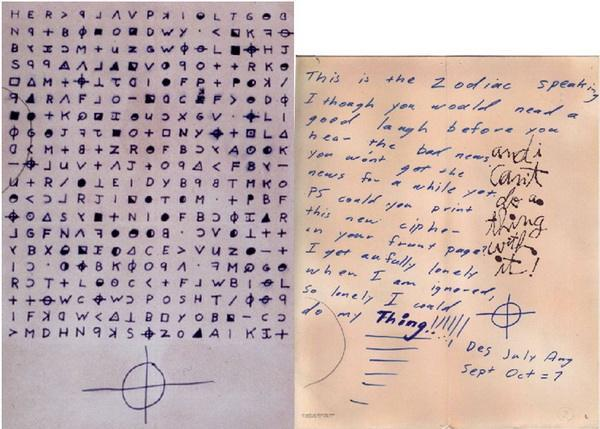## 计蒜客 – 情报加密

1162 0 2020年3月20日

0 0 投票数

2
A
DB
DBAADB

4

int len = strlen(ch + 1);
for (int i = 1; i <= len; i++) {
for (int p = 0; p < tot; p++) {
if (sta[p]) {
// 如果当前节点是一个关键情报，则不能走这条路
continue;
}
for (int x = 0; x < MAXC; x++) {
if (sta[child[p][x]]) {
// 如果走下去的方向是关键情报，则需要换一条路
continue;
}
// 到这里，就是一个安全的路，可以做一次状态转移
// 如果走过去的那个方向，与情报中下一个字符是一样的，就不需要一次修改
int modify = ch[i] == 'a' + x ? 0 : 1;
dp[i + 1][child[p][x]] = min(dp[i + 1][child[p][x]], dp[i][p] + modify);
}
}
}

int solve(char *ch) {
cout << strlen(ch + 1) << endl;

// 初始化 dp 数组
memset(dp, 0x3F, sizeof(dp));
dp = 0; // 字符串下标从 1 开始

int len = strlen(ch + 1);
for (int i = 1; i <= len; i++) {
for (int p = 0; p < tot; p++) {
if (sta[p] || dp[i][p] == INF) {
// 如果当前节点是一个关键情报，则不能走这条路
continue;
}
for (int x = 0; x < MAXC; x++) {
if (sta[child[p][x]]) {
// 如果走下去的方向是关键情报，则需要换一条路
continue;
}
// 到这里，就是一个安全的路，可以做一次状态转移
// 如果走过去的那个方向，与情报中下一个字符是一样的，就不需要一次修改
int modify = ch[i] == 'A' + x ? 0 : 1;
dp[i + 1][child[p][x]] = min(dp[i + 1][child[p][x]], dp[i][p] + modify);
}
}
}

int ans = INF;
for (int i = 0; i < tot; i++) {
// 注意是 len + 1
ans = min(ans, dp[len + 1][i]);
}
return ans == INF ? -1 : ans;
}

if (sta[fail[p]]) {
sta[p] = 1;
}
// 即 sta[p] |= sta[fail[p]]

#include <bits/stdc++.h>

using namespace std;

const int MAXC = 4; // 这里字符范围只有 A ~ D
const int MAXN = 1007;
const int INF = 0x3F3F3F3F;

int child[MAXN][MAXC], fail[MAXN], sta[MAXN], Q[MAXN];
int tot;
int dp[MAXN][MAXN];

/**
* AC 自动机
*/
struct AC_Automaton {

/**
* 清空
*/
void clear() {
memset(child, 255, sizeof(child));
memset(fail, 0, sizeof(fail));
tot = 0;
memset(sta, 0, sizeof(sta));
}

/**
* 插入单词
* @param ch 单词，该单词下标从 1 开始
*/
void insert(char *ch) {
int p = 0, l = strlen(ch + 1);
for (int i = 1; i <= l; i++) {
if (child[p][ch[i] - 'A'] == -1) child[p][ch[i] - 'A'] = ++tot;
p = child[p][ch[i] - 'A'];
}
sta[p] = 1; // 以结点 p 的字符串是否存在，由于本题只需要是否存在，设置 true 就好了，像上一题还需要统计个数，可以改为 sta[p]++，表示有多少个
}

/**
* 对插入了单词的前缀树构造失败指针
*/
void build() {
int l = 0, r = 0;
for (int i = 0; i < MAXC; i++)
if (child[i] == -1)
child[i] = 0;
else
Q[++r] = child[i];
while (l < r) {
int p = Q[++l];
if (sta[fail[p]]) {
sta[p] = 1;
}
for (int i = 0; i < MAXC; i++)
if (child[p][i] == -1)
child[p][i] = child[fail[p]][i];
else {
fail[child[p][i]] = child[fail[p]][i];
Q[++r] = child[p][i];
}
}
}

/**
*
* @param ch 字符串，下标从 1 开始
* @return 最小修改次数
*/
int solve(char *ch) {

// 初始化 dp 数组
memset(dp, 0x3F, sizeof(dp));
dp = 0; // 字符串下标从 1 开始

int len = strlen(ch + 1);
for (int i = 1; i <= len; i++) {
for (int p = 0; p < tot; p++) {
if (sta[p] || dp[i][p] == INF) {
// 如果当前节点是一个关键情报，则不能走这条路
continue;
}
for (int x = 0; x < MAXC; x++) {
if (sta[child[p][x]]) {
// 如果走下去的方向是关键情报，则需要换一条路
continue;
}
// 到这里，就是一个安全的路，可以做一次状态转移
// 如果走过去的那个方向，与情报中下一个字符是一样的，就不需要一次修改
int modify = ch[i] == 'A' + x ? 0 : 1;
dp[i + 1][child[p][x]] = min(dp[i + 1][child[p][x]], dp[i][p] + modify);
}
}
}

int ans = INF;
for (int i = 0; i < tot; i++) {
// 注意是 len + 1
ans = min(ans, dp[len + 1][i]);
}
return ans == INF ? -1 : ans;
}
} T;

int main() {
// 构造 AC 自动机
auto ac = new AC_Automaton();
ac->clear();
int n;
scanf("%d", &n);
// 读入单词，加入前缀树
char *s = (char *) malloc(sizeof(char) * MAXN);
for (int i = 0; i < n; i++) {
// 字符串下标从 1 开始
scanf("%s", s + 1);
ac->insert(s);
}
// 根据前缀树中的单词构造失败指针，即构造字典
ac->build();
// 给定的文章，下标从 1 开始
char *t = (char *) malloc(sizeof(char) * MAXN);
scanf("%s", t + 1);
// 执行操作，输出结果
printf("%d\n", ac->solve(t));
return 0;
}
0 0 投票数（可选）如果您也有个人网站，不妨分享一下

0 评论

Warning: error_log(/home/wwwroot/www.jxtxzzw.com/wp-content/plugins/spider-analyser/#log/log-0813.txt): failed to open stream: No such file or directory in /home/wwwroot/www.jxtxzzw.com/wp-content/plugins/spider-analyser/spider.class.php on line 2900